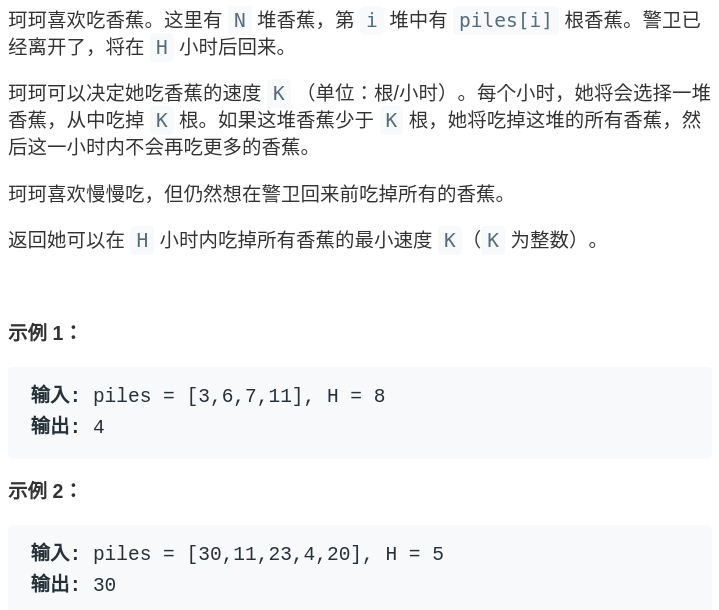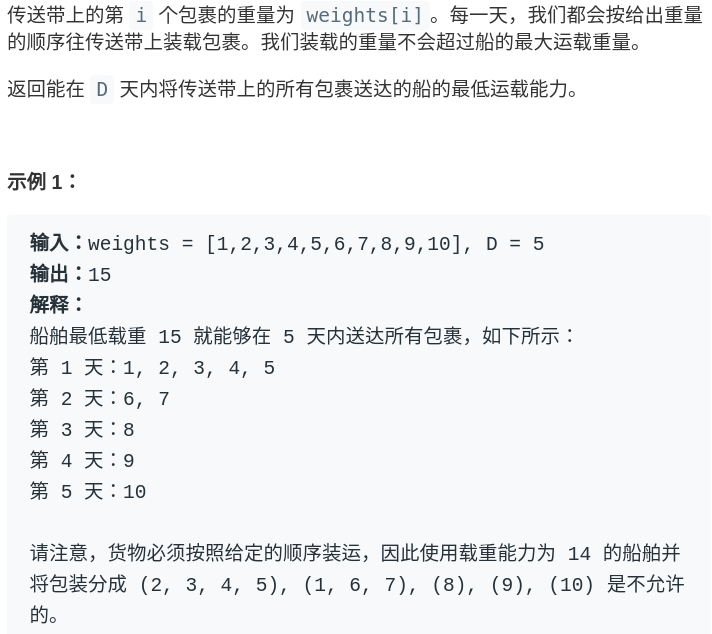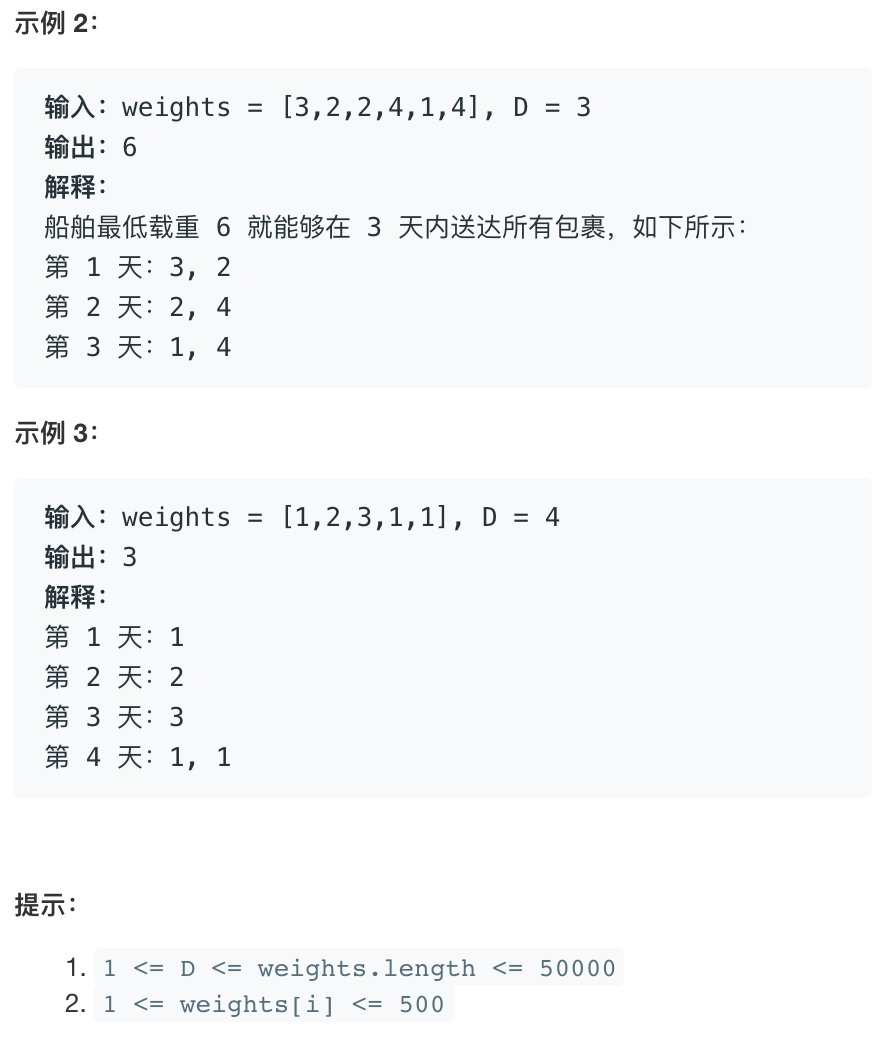• 累计撰写 270 篇文章
• 累计创建 6 个标签
• 累计收到 1 条评论

### 目 录CONTENT# 二分查找的应用.md2021-08-22 / 0 评论 / 0 点赞 / 8 阅读 / 2,460 字 / 正在检测是否收录...

@

## 一、题目描述：爱吃香蕉的珂珂

https://leetcode.cn/problems/koko-eating-bananas/## 二、分析

• 也就是说，Koko 每小时最多吃一堆香蕉，如果吃不下的话留到下一小时再吃；
• 如果吃完了这一堆还有胃口，也只会等到下一小时才会吃下一堆。在这个条件下，让我们确定 Koko 吃香蕉的最小速度（根/小时）。
• 如果直接给你这个情景，你能想到哪里能用到二分查找算法吗？如果没有见过类似的问题，恐怕是很难把这个问题和二分查找联系起来的。
• 那么我们先抛开二分查找技巧，想想如何暴力解决这个问题呢？
• 首先，算法要求的是「H 小时内吃完香蕉的最小速度」，我们不妨称为 speed，请问 speed 最大可能为多少，最少可能为多少呢？
• 显然最少为 1，最大为 max(piles)，因为一小时最多只能吃一堆香蕉。那么暴力解法就很简单了，只要从 1 开始穷举到 max(piles)，一旦发现发现某个值可以在 H 小时内吃完所有香蕉，这个值就是最小速度
int minEatingSpeed(int[] piles, int H)
{
// piles 数组的最大值
int max = getMax(piles);
for (int speed = 1; speed < max; speed++)
{
// 以 speed 是否能在 H 小时内吃完香蕉
if (canFinish(piles, speed, H))
return speed;
}
return max;
}

• 注意这个 for循环，就是在连续的空间线性搜索，这就是二分查找可以发挥作用的标志。由于我们要求的是最小速度，所以可以用一个搜索左侧边界的二分查找来代替线性搜索，提升效率
int minEatingSpeed(int[] piles, int H)
{
// 套用搜索左侧边界的算法框架
int left = 1, right = getMax(piles) + 1;
while (left < right)
{
// 防止溢出
int mid = left + (right - left) / 2;
if (canFinish(piles, mid, H))
{
right = mid;
}
else
{
left = mid + 1;
}
}
return left;
}


// 时间复杂度 O(N)
boolean canFinish(int[] piles, int speed, int H)
{
int time = 0;
for (int n : piles)
{
time += timeOf(n, speed);
}
return time <= H;
}

int timeOf(int n, int speed)
{
return (n / speed) + ((n % speed > 0) ? 1 : 0);
}

int getMax(int[] piles)
{
int max = 0;
for (int n : piles)
max = Math.max(n, max);
return max;
}


## 三、完整代码

class Solution {
public:
// 时间复杂度 O(N)
bool canFinish(vector<int> piles, int speed, int H)
{
int time = 0;
for (int n : piles)
{
time += timeOf(n, speed);
}
return time <= H;
}

int timeOf(int n, int speed)
{
return (n / speed) + ((n % speed > 0) ? 1 : 0);
}

int getMax(vector<int> piles)
{
int maxx = 0;
for (int n : piles)
maxx = std::max(n, maxx);
return maxx;
}

int minEatingSpeed(vector<int>& piles, int H) {
// 套用搜索左侧边界的算法框架
int left = 1, right = getMax(piles) + 1;
while (left < right)
{
// 防止溢出
int mid = left + (right - left) / 2;
if (canFinish(piles, mid, H))
{
right = mid;
}
else
{
left = mid + 1;
}
}
return left;
}
};


## 四、问题描述：在 D 天内送达包裹的能力

https://leetcode.cn/problems/capacity-to-ship-packages-within-d-days/## 五、分析

• 要在 D 天内运输完所有货物，货物不可分割，如何确定运输的最小载重呢（下文称为 cap）？
• 其实本质上和 Koko 吃香蕉的问题一样的，首先确定 cap 的最小值和最大值分别为 max(weights) 和 sum(weights)
• 我们要求最小载重，所以可以用搜索左侧边界的二分查找算法优化线性搜索：

## 六、代码

// 寻找左侧边界的二分查找
int shipWithinDays(int[] weights, int D)
{
// 载重可能的最小值
int left = getMax(weights);

// 载重可能的最大值 + 1
int right = getSum(weights) + 1;
while (left < right)
{
int mid = left + (right - left) / 2;
if (canFinish(weights, D, mid))
{
right = mid;
}
else
{
left = mid + 1;
}
}
return left;
}

// 如果载重为 cap，是否能在 D 天内运完货物？
boolean canFinish(int[] w, int D, int cap)
{
int i = 0;
for (int day = 0; day < D; day++)
{
int maxCap = cap;
while ((maxCap -= w[i]) >= 0)
{
i++;
if (i == w.length)
return true;
}
}
return false;
}

0